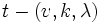# Block design

Jump to: navigation, search

## Definition

### Definition with symbols

A$(v,k,\lambda)$-block design is the following data:

• A set$S$ with$v$ elements, called the vertices
• A collection of$k$-element subsets of$S$, called blocks. The number of blocks is denoted as$b$

Satisfying the following conditions:

• Every point is contained in the same number of blocks (this is denoted as$r$)
• Given any two points, the number of blocks containing both of them is$\lambda$
Note that$b$ and$r$ are dependent on the other three parameters via some obvious relations PLACEHOLDER FOR INFORMATION TO BE FILLED IN: [SHOW MORE]

This is also called a BIBD or a Balanced Incomplete Block Design.

### Further definition

A generalization of block design is to$t$-block design. A$t$-block design has the property that for any$t$ elements, the number of blocks cotaining all$t$ of them is fixed and independent of the choice of elements. Such a thing is called, in more detail, a$t-(v,k,\lambda)$ block design.